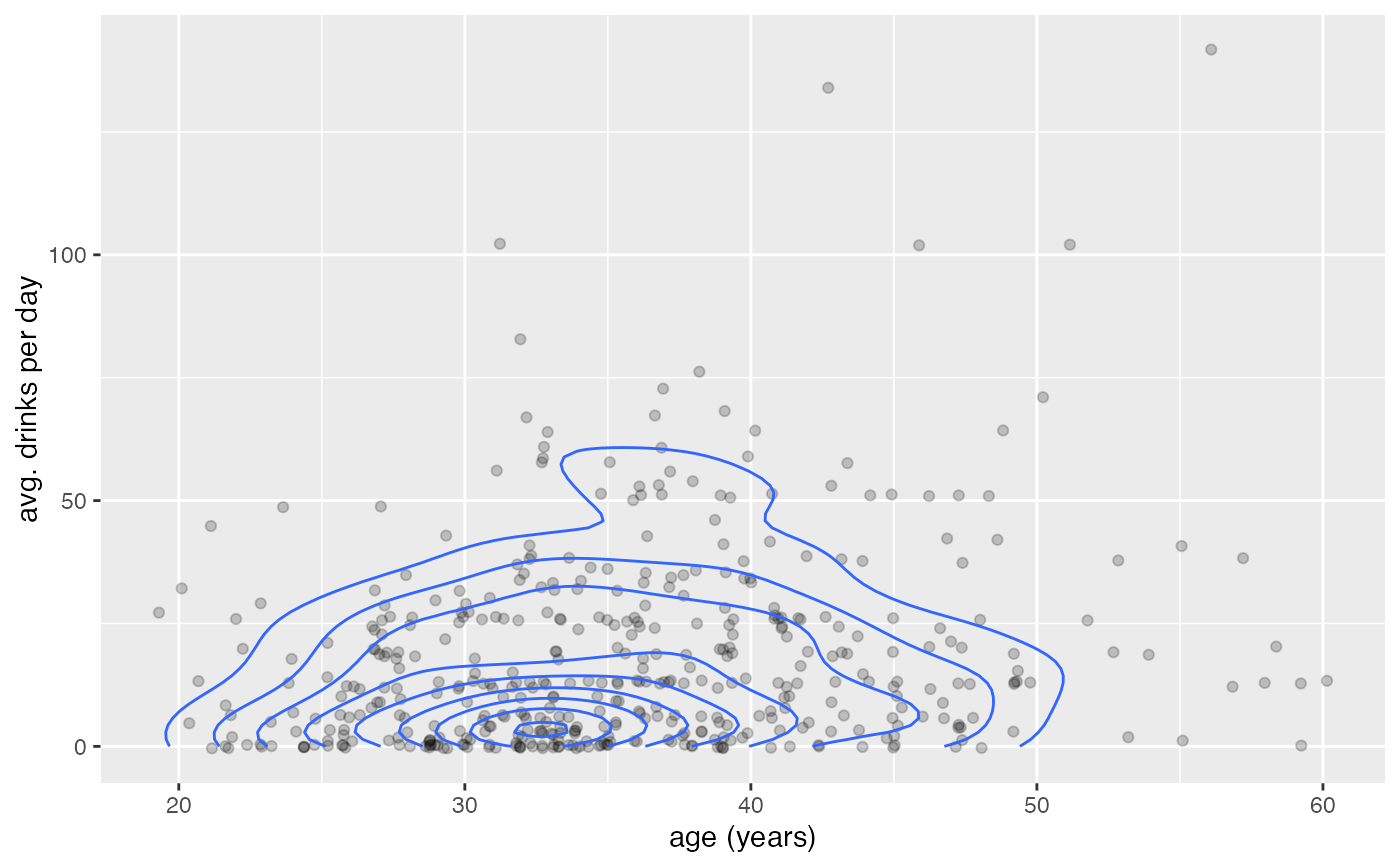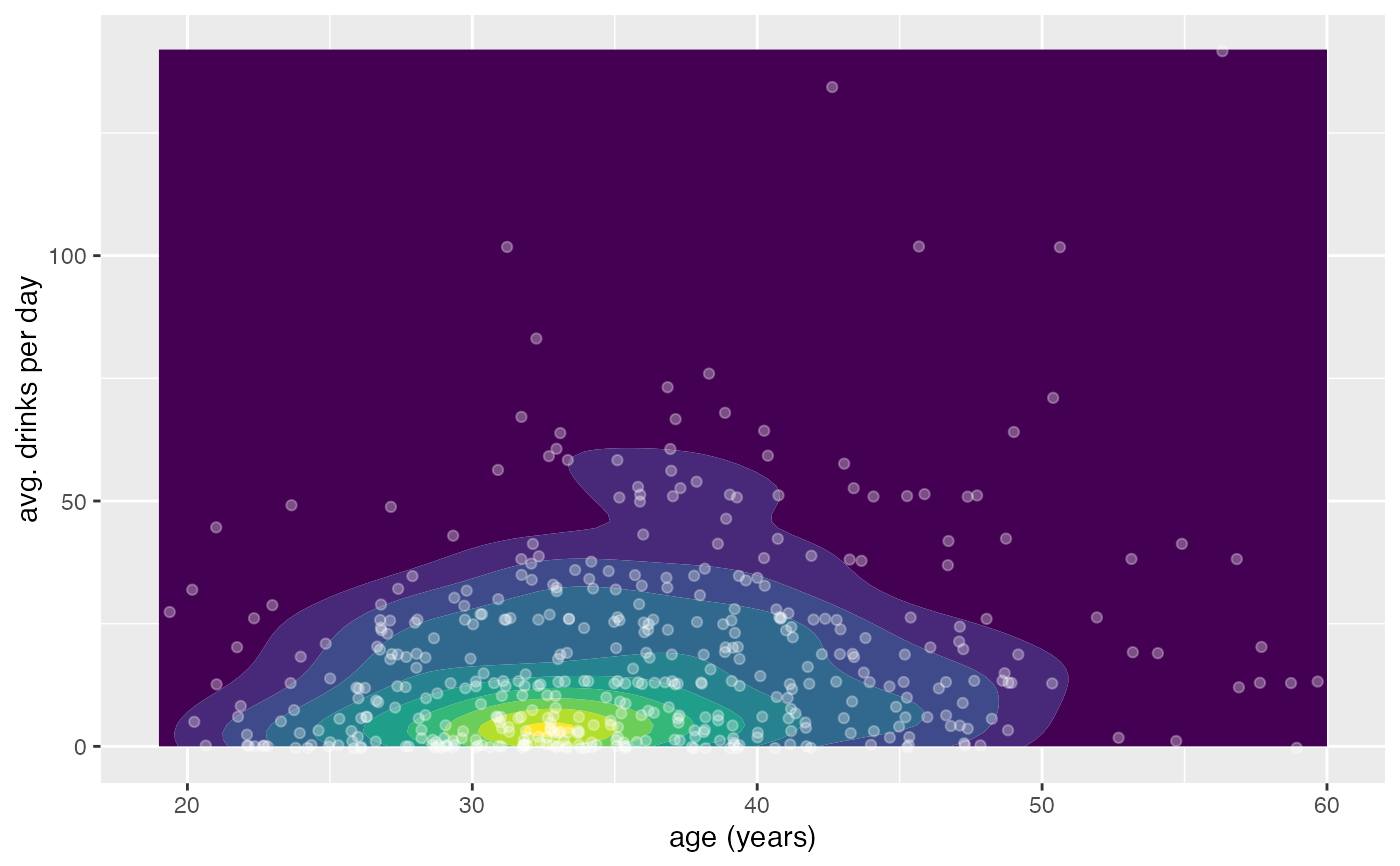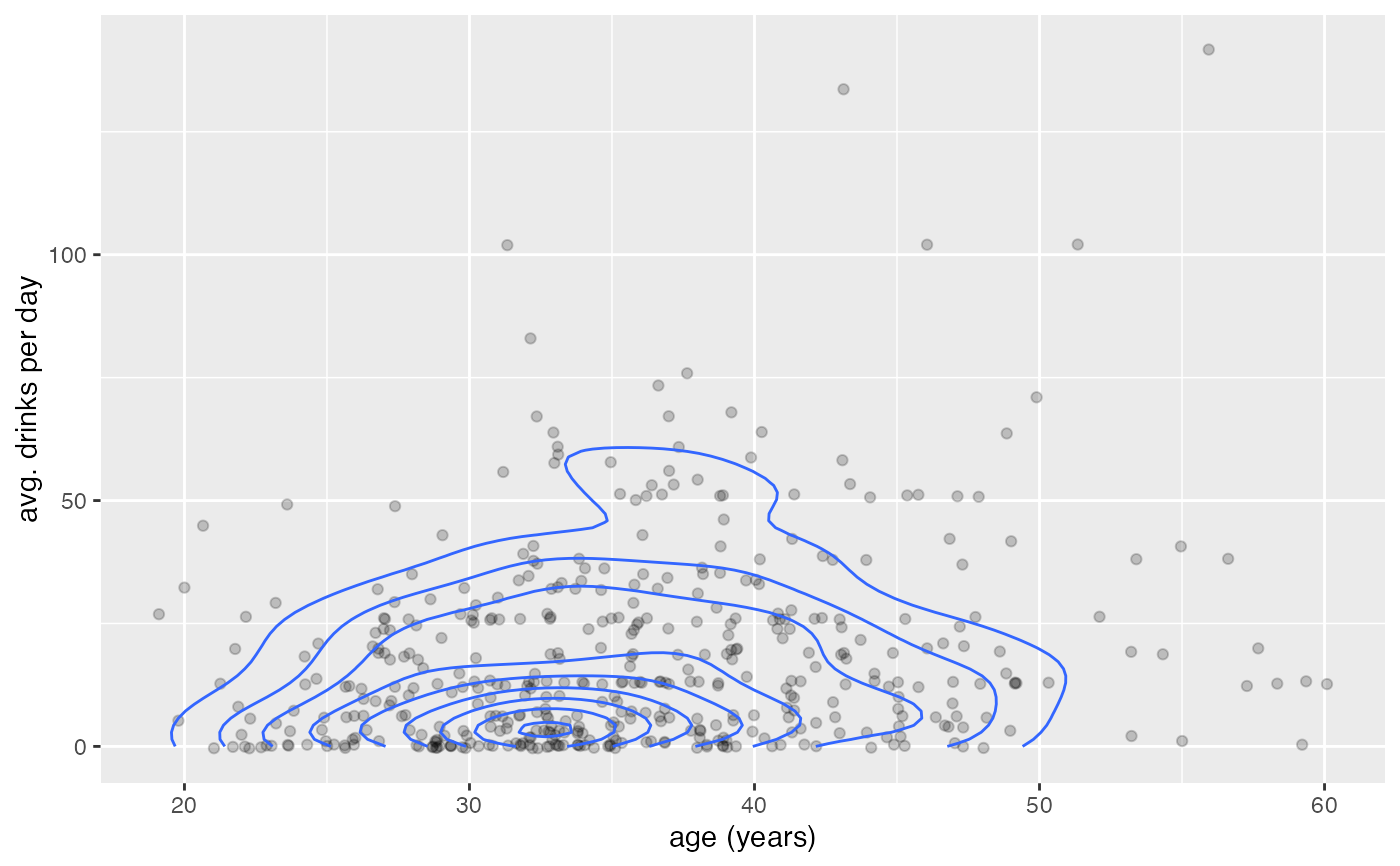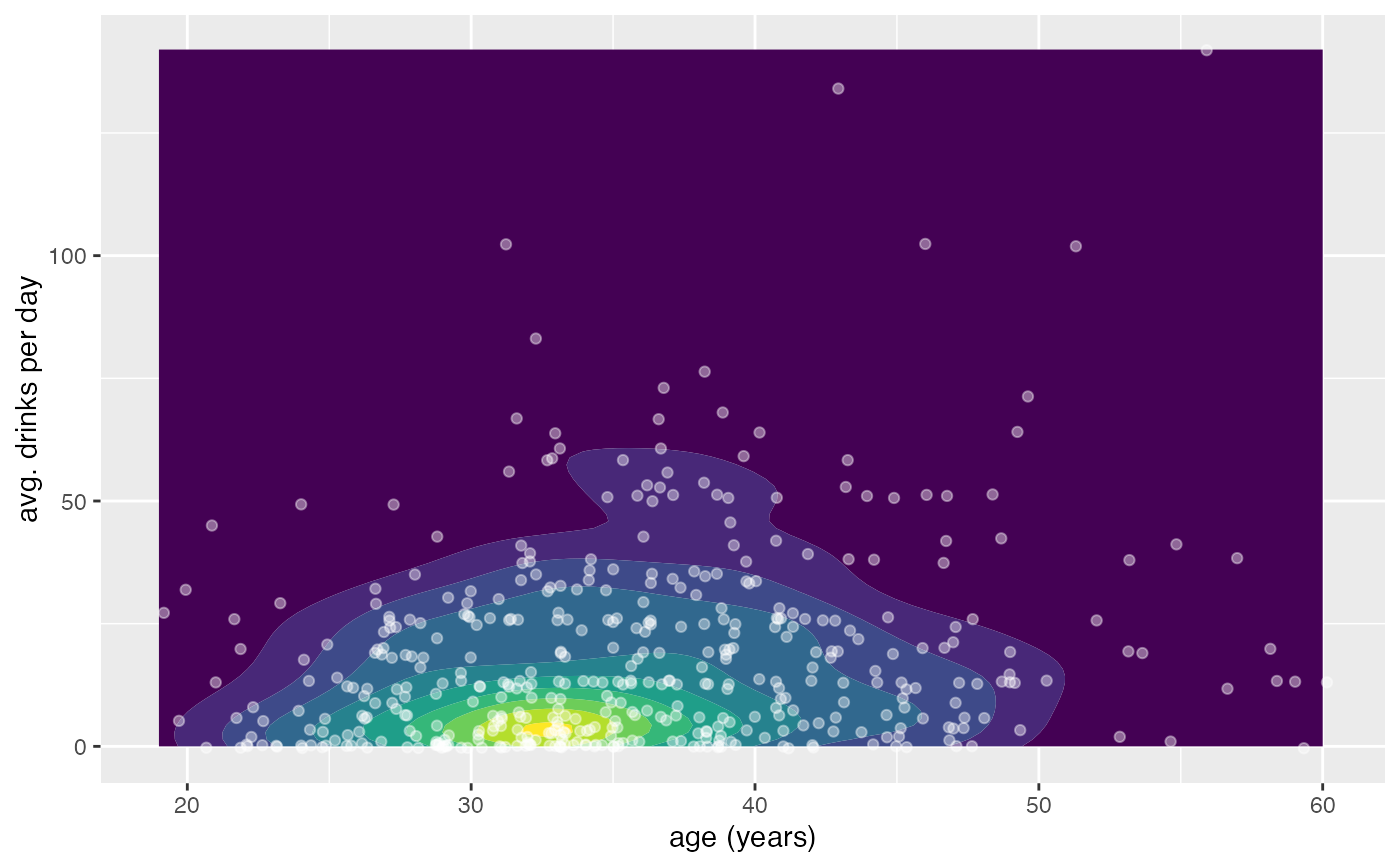Perform a 2D kernel density estimation using MASS::kde2d() and display the results with contours. This can be useful for dealing with overplotting. This is a 2D version of geom_density(). geom_density_2d() draws contour lines, and geom_density_2d_filled() draws filled contour bands.

gf_density_2d(
object = NULL,
gformula = NULL,
data = NULL,
...,
alpha,
color,
group,
linetype,
size,
contour = TRUE,
n = 100,
h = NULL,
lineend = "butt",
linejoin = "round",
linemitre = 1,
xlab,
ylab,
title,
subtitle,
caption,
geom = "density_2d",
stat = "density_2d",
position = "identity",
show.legend = NA,
show.help = NULL,
inherit = TRUE,
environment = parent.frame()
)

gf_density_2d_filled(
object = NULL,
gformula = NULL,
data = NULL,
...,
alpha,
color,
group,
linetype,
size,
contour = TRUE,
n = 100,
h = NULL,
lineend = "butt",
linejoin = "round",
linemitre = 1,
xlab,
ylab,
title,
subtitle,
caption,
geom = "density_2d_filled",
stat = "density_2d_filled",
position = "identity",
show.legend = NA,
show.help = NULL,
inherit = TRUE,
environment = parent.frame()
)

gf_density2d(
object = NULL,
gformula = NULL,
data = NULL,
...,
alpha,
color,
group,
linetype,
size,
contour = TRUE,
n = 100,
h = NULL,
lineend = "butt",
linejoin = "round",
linemitre = 1,
xlab,
ylab,
title,
subtitle,
caption,
geom = "density2d",
stat = "density2d",
position = "identity",
show.legend = NA,
show.help = NULL,
inherit = TRUE,
environment = parent.frame()
)

gf_density2d_filled(
object = NULL,
gformula = NULL,
data = NULL,
...,
alpha,
color,
group,
linetype,
size,
contour = TRUE,
n = 100,
h = NULL,
lineend = "butt",
linejoin = "round",
linemitre = 1,
xlab,
ylab,
title,
subtitle,
caption,
geom = "density2d_filled",
stat = "density_2d_filled",
position = "identity",
show.legend = NA,
show.help = NULL,
inherit = TRUE,
environment = parent.frame()
)

## Arguments

object When chaining, this holds an object produced in the earlier portions of the chain. Most users can safely ignore this argument. See details and examples. A formula with shape y ~ x. Faceting can be achieved by including | in the formula. The data to be displayed in this layer. There are three options: If NULL, the default, the data is inherited from the plot data as specified in the call to ggplot(). A data.frame, or other object, will override the plot data. All objects will be fortified to produce a data frame. See fortify() for which variables will be created. A function will be called with a single argument, the plot data. The return value must be a data.frame, and will be used as the layer data. A function can be created from a formula (e.g. ~ head(.x, 10)). Additional arguments. Typically these are (a) ggplot2 aesthetics to be set with attribute = value, (b) ggplot2 aesthetics to be mapped with attribute = ~ expression, or (c) attributes of the layer as a whole, which are set with attribute = value. Opacity (0 = invisible, 1 = opaque). A color or a formula used for mapping color. Used for grouping. A linetype (numeric or "dashed", "dotted", etc.) or a formula used for mapping linetype. A numeric size or a formula used for mapping size. If TRUE, contour the results of the 2d density estimation. Number of grid points in each direction. Bandwidth (vector of length two). If NULL, estimated using MASS::bandwidth.nrd(). Line end style (round, butt, square). Line join style (round, mitre, bevel). Line mitre limit (number greater than 1). Label for x-axis. See also gf_labs(). Label for y-axis. See also gf_labs(). Title, sub-title, and caption for the plot. See also gf_labs(). Title, sub-title, and caption for the plot. See also gf_labs(). Title, sub-title, and caption for the plot. See also gf_labs(). Use to override the default connection between geom_density_2d and stat_density_2d. Use to override the default connection between geom_density_2d and stat_density_2d. Position adjustment, either as a string, or the result of a call to a position adjustment function. logical. Should this layer be included in the legends? NA, the default, includes if any aesthetics are mapped. FALSE never includes, and TRUE always includes. It can also be a named logical vector to finely select the aesthetics to display. If TRUE, display some minimal help. A logical indicating whether default attributes are inherited. An environment in which to look for variables not found in data.

a gg object

## Specifying plot attributes

Positional attributes (a.k.a, aesthetics) are specified using the formula in gformula. Setting and mapping of additional attributes can be done through the use of additional arguments. Attributes can be set can be set using arguments of the form attribute = value or mapped using arguments of the form attribute = ~ expression.

In formulas of the form A | B, B will be used to form facets using facet_wrap() or facet_grid(). This provides an alternative to gf_facet_wrap() and gf_facet_grid() that is terser and may feel more familiar to users of lattice.

## Evaluation

Evaluation of the ggplot2 code occurs in the environment of gformula. This will typically do the right thing when formulas are created on the fly, but might not be the right thing if formulas created in one environment are used to create plots in another.

ggplot2::geom_density_2d()

## Examples

gf_jitter(avg_drinks ~ age,
alpha = 0.2, data = mosaicData::HELPrct,
width = 0.4, height = 0.4
) %>%
gf_density_2d(avg_drinks ~ age, data = mosaicData::HELPrct)gf_density_2d_filled(avg_drinks ~ age, data = mosaicData::HELPrct, show.legend = FALSE) %>%
gf_jitter(avg_drinks ~ age,
alpha = 0.3, data = mosaicData::HELPrct,
width = 0.4, height = 0.4,
color = "white"
)gf_jitter(avg_drinks ~ age,
alpha = 0.2, data = mosaicData::HELPrct,
width = 0.4, height = 0.4
) %>%
gf_density2d(avg_drinks ~ age, data = mosaicData::HELPrct)gf_density2d_filled(avg_drinks ~ age, data = mosaicData::HELPrct, show.legend = FALSE) %>%
gf_jitter(avg_drinks ~ age,
alpha = 0.4, data = mosaicData::HELPrct,
width = 0.4, height = 0.4,
color = "white"
)